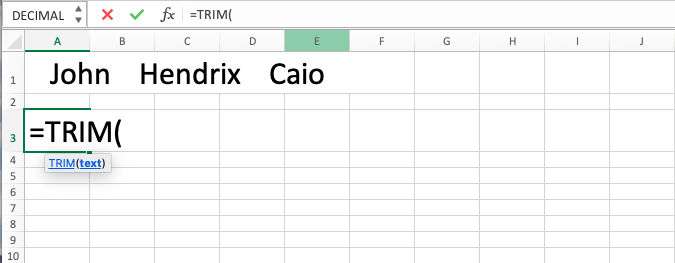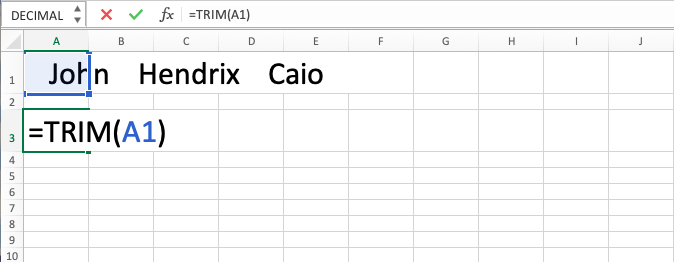TRIM Excel Function - Compute Expert

# TRIM Excel Function

In this tutorial, you will learn TRIM in excel formula. TRIM in excel formula is useful to remove redundant spaces from a text that you have in the spreadsheet.

## Why do We Need to Learn TRIM in Excel Formula?

In doing processing in the spreadsheet, sometimes we get data from another source to be saved in our file. The problem is data from other sources is often not “clean” and one of the most common problems is the redundant spaces in each of the data. This can give a bad impact on the data processing that we want to do using formula or other functions in the spreadsheet. The problem is because the formula or other functions do not think the data with and without the spaces are the same. They think that those data are different and that becomes a problem when we want to use the data for our processing in the spreadsheet.

For example to illustrate the problem, imagine if we are doing criteria based processing for the numbers that we got from an online database source of our company. When we want to do the calculation, often the texts of the data from another source that we want to use as the basis of criteria evaluation for the calculation is not “clean” and has redundant spaces in it. Thus, the spreadsheet thinks of them as text data which does not fit the criteria. This can give effect in the wrong result of the formula that we want to use to make the criteria based calculation process easier.

Or when we want to use the entries from our online form and we want to mark the cells with particular entries using conditional formatting. But because there are unneeded spaces that are often found in the “not clean” entries from external sources, then our condition in the conditional formatting can produce FALSE even though the cell contains the entry that we require. Hence, the cell marks that we want in the spreadsheet are not correct and not based on our requirements.

To answer this problem and do data cleaning to remove the unwanted spaces, then you can use the TRIM excel function. TRIM in excel formula can remove the unneeded spaces in a text as per your desire in doing the cleaning process. It is very useful if you often process data sourced from outside of your spreadsheet and need cleaning process on them before they can be used and analyzed correctly.

## What is TRIM Excel Function?

TRIM excel function is a function with the usage objective to clean a text from redundant spaces. TRIM excel function will remove all but one space between texts and all the spaces in the front and back.

Briefly, TRIM in excel formula’s input can be explained as follows:

=TRIM(text)

Note:
text = the text that you want to remove the redundant spaces from

## How to Use TRIM in Excel Formula?

The following will explain the writing of the TRIM excel function. TRIM in excel formula is easy and simple to use because it only needs the input of the data that you want to clean up for your needs.

## Using TRIM Excel Function

1. Type equal sign ( = ) in the cell where you want to put the result in2. Type TRIM (can be with large and small letters) and open bracket sign after =3. Type text or cell coordinate where the text which you want to clean the spaces is in after open bracket sign. If you want to type it directly without the use of a cell coordinate, then do not forget to type it between two quotes signs (“”)4. Type close bracket sign5. Press Enter
6. The process is done!## Exercise

After you have learned how to use TRIM in excel formula through the detailed tutorial above, now is the time you sharpen your understanding by doing the following exercise!

### Questions

1. Clean the text on the left!
2. Is the text in the left “John”? Use IF and produce V if it is “John” by using TRIM excel function first for the text so the result is correct! Produce X if it is not “John”!
3. Use IF to sum the number in column C if the text in column B is “V”! Remove the redundant spaces in the text first so the formula can function well!

• TRIM in excel formula can only remove spaces from ASCII characters. It will not remove things like non-breaking spaces from Html code
• If you want to remove non-print characters too from your text, then you can combine TRIM excel function with CLEAN formula

Get updated excel info from Compute Expert by registering your email. It's free!